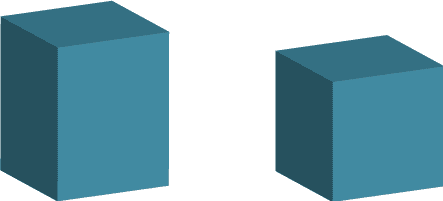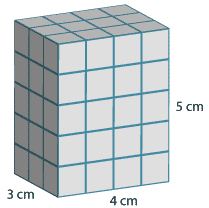## Content description

Connect volume and capacity and their units of measurement (ACMMG138)

Source: Australian Curriculum, Assessment and Reporting Authority (ACARA)

### Volume of rectangular prisms

A rectangular prism is a three-dimensional object with rectangles as all of its faces. If all the faces of the prism are squares then the rectangular prism is a cube.The volume of a rectangular prism is a measure of the space inside the prism.If we cut this rectangular prism up into cubes, with each cube having a volume of one cubic centimetre, then there are 4 × 3 × 5 = 60 cubes. The volume of each cube is one cubic centimetre so the volume of the rectangular prism above is 60 cubic centimetres, or 60 cm3.

The side lengths of a rectangular prism are generally called the length, the width and the height. The volume of any rectangular prism is given by:

\begin{align}\text{Volume of a rectangular prism} &= \text{length}\ × \text{width}\ × \text{height}\\\\ &=lwh\end{align}

If we take the side lengths in the example above to be 4 cm, 3 cm and 5 cm, then we calculate its volume as follows:

\begin{align}\text{Volume}&=lwh\\\\ &=4×3×5\\\\ &=60\ \text{cm}^3\end{align}

We can also calculate volume in cubic millimetres or cubic metres.